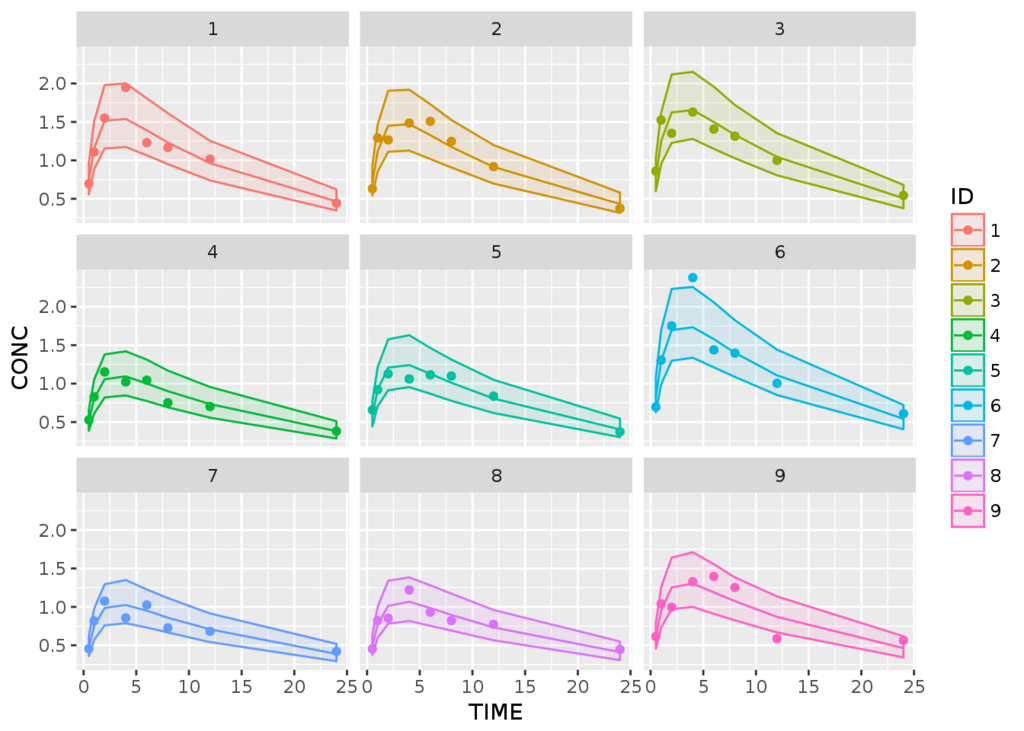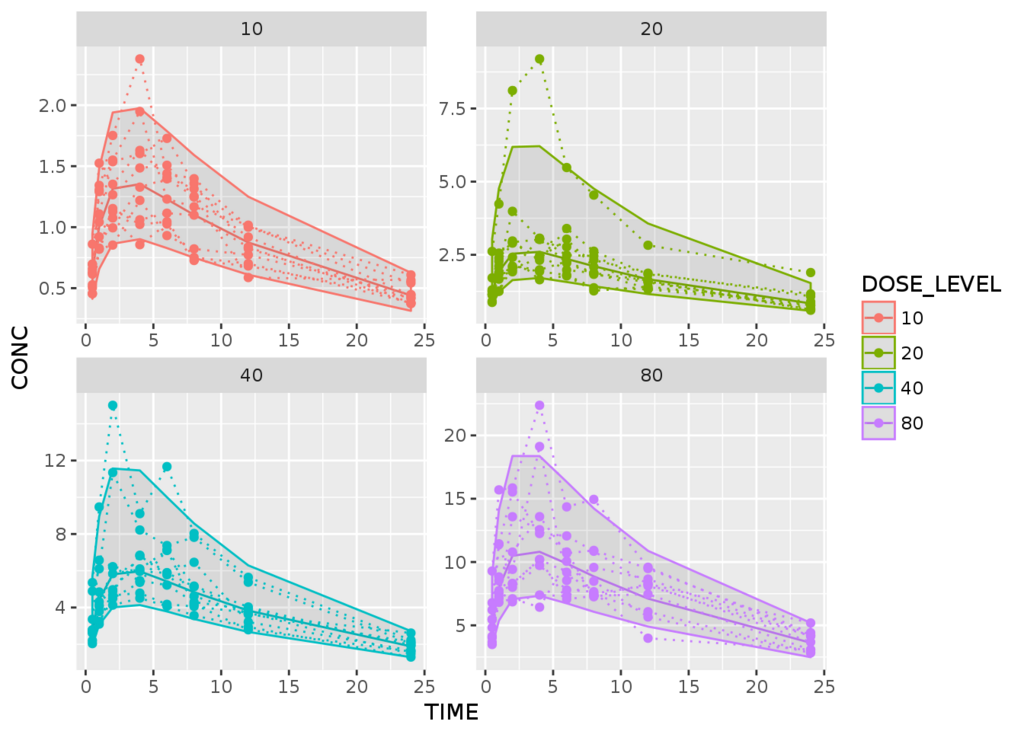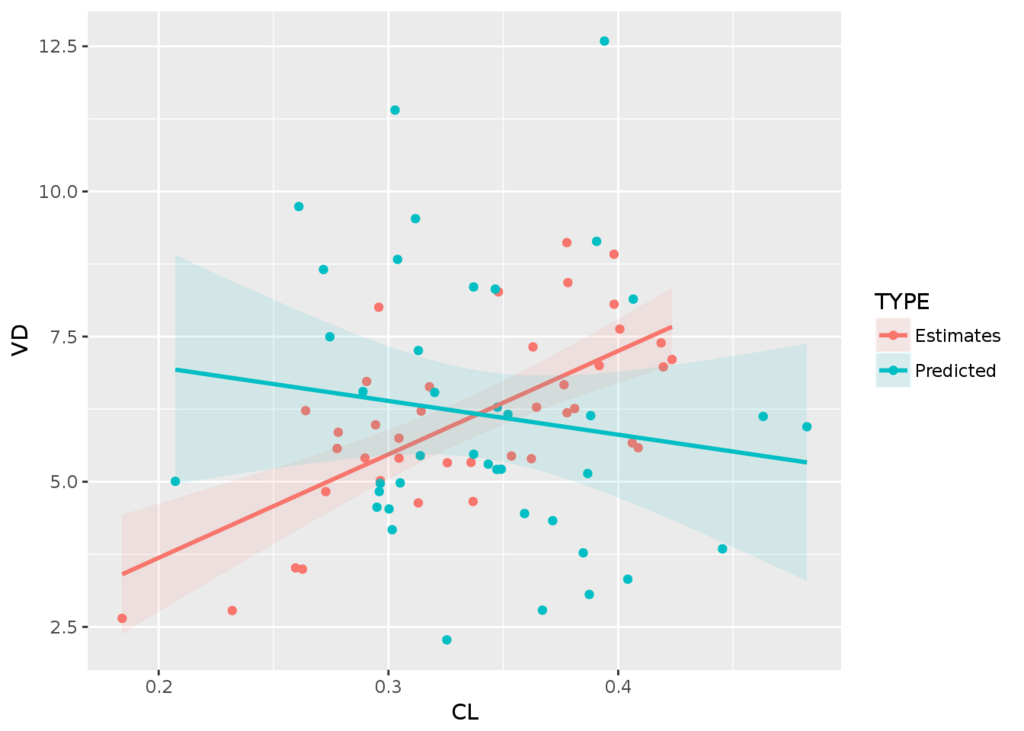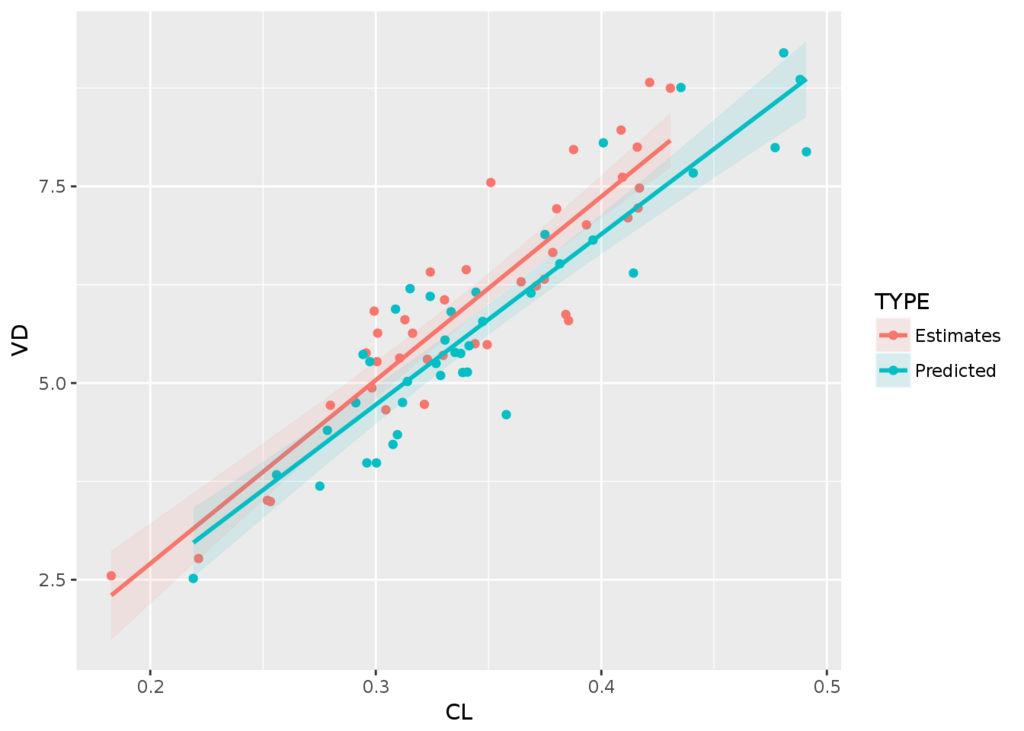# Simulating parametric survival model with parametric bootstrap to capture uncertainty

I recently released an R package on CRAN called`survParamSim` for parametric survival simulation, and here want to describe a bit more on details & motivations behind developing this package.

Parametric Survival Simulation with Parameter Uncertainty • survParamSim

The purpose of `survParamSim` is to package the R functions developed by a great scientist, the late Laurent Claret, and streamline the function definition/APIs to make these widely available to the public. Laurent developed these functions for his pioneering works in applying tumor growth inhibition models for clinical outcome predictions of oncology studies, such as the one below. clincancerres.aacrjournals.org

Explaining the theory behind his works is well beyond the scope of this short blog post, but the key technical highlight is that we are using parametric survival models with various covariates for predicting survival profiles and treatment benefits. In applying these workflows in the context of drug development (or maybe in most of other contexts), we want to make a prediction of not only the mean survival profiles but also the uncertainties associated with model predictions.

There are mainly two sources of uncertainty for such survival predictions. The first one is due to the nature of the survival prediction: because survival (or rather failure) is modeled as probabilistic processes, a predicted survival curve for a group will never be identical after multiple simulations. There is another source of uncertainty, which is the uncertainty associated with model parameters, such as coefficients associated with covariates in the model.

The first element is fairly straightforward to evaluate - we can run simulations many times and quantify how variable the survival curves can be by looking at prediction intervals. For example, we expect the prediction interval to generally get narrower if we make a prediction with larger number of patients. However, for the second part, there is not a readily available method for exploration to my (limited) knowledge. `survival::predict.survreg()` has `se.fit` option, but it is not straightforward to carry the output from this option for making predicted survival profiles of a group.

These are the main reasons for putting together this package - to enable the incorporation of above two sources of uncertainty in survival predictions, using parametric models obtained with `survival::survreg()`. For the second element of the uncertainty, we are using parametric bootstrap from variance-covariance matrix of the model parameter estimates.

# Example

The code below is essentially the same as the one on GitHub pages, just showing a simple work flow to give you a sense of what to expect in terms of outputs. Vignette here has a bit more explanations on the workflow.

For this example, we use `colon` dataset in `survival` package, where the treatment benefit of Levamisole+5-FU was compared with control (called as Obs). Various covariates were further explored, such as extent of tumor local spread.

First, you would run `survreg` to fit parametric survival model:

```library(tidyverse)
library(survival)
library(survParamSim)

set.seed(12345)

# ref for dataset https://vincentarelbundock.github.io/Rdatasets/doc/survival/colon.html
colon2 <-
as_tibble(colon) %>%
# recurrence only and not including Lev alone arm
filter(rx != "Lev", etype == 1) %>%
# Same definition as Lin et al, 1994
mutate(rx = factor(rx, levels = c("Obs", "Lev+5FU")),
depth = as.numeric(extent <= 2))```

Here we have two covariates, `node4` and `depth`, in addition to the treatment assignment `rx`.

```fit.colon <- survreg(Surv(time, status) ~ rx + node4 + depth,
data = colon2, dist = "lognormal")```

Next, parametric bootstrap simulation can be run with `surv_param_sim()` function from this package:

```sim <-
surv_param_sim(object = fit.colon, newdata = colon2,
censor.dur = c(1800, 3000),
# Simulating only 100 times to make the example go fast
n.rep = 100)```

The output object merely has raw simulated survival data with 100 repetition (defined by `n.rep` argument). You can find what options you have on this output by simply printing the object.

```sim
#> ---- Simulated survival data with the following model ----
#> survreg(formula = Surv(time, status) ~ rx + node4 + depth, data = colon2,
#>     dist = "lognormal")
#>
#> * Use `extract_sim()` function to extract individual simulated survivals
#> * Use `calc_km_pi()` function to get survival curves and median survival time
#> * Use `calc_hr_pi()` function to get hazard ratio
#>
#> * Settings:
#>     #simulations: 100
#>     #subjects: 619 (without NA in model variables)```

To visualize simulated survival curves with uncertainly, you can use `calc_km_pi()` and `plot_km_pi()` functions sequentially. You can also specify faceting/grouping with `trt` and `group` input arguments.

```km.pi <- calc_km_pi(sim, trt = "rx", group = c("node4", "depth"))

km.pi
#> ---- Simulated and observed (if calculated) survival curves ----
#> * Use `extract_median_surv()` to extract median survival times
#> * Use `extract_km_pi()` to extract prediction intervals of K-M curves
#> * Use `plot_km_pi()` to draw survival curves
#>
#> * Settings:
#>     trt: rx
#>     group: node4
#>     pi.range: 0.95
#>     calc.obs: TRUE

plot_km_pi(km.pi) +
theme(legend.position = "bottom") +
labs(y = "Recurrence free rate") +
expand_limits(y = 0)```

Similarly, you can visualize hazard ratios and prediction intervals with `calc_hr_pi()` and `plot_hr_pi()` functions.

```hr.pi <- calc_hr_pi(sim, trt = "rx", group = c("depth"))

hr.pi
#> ---- Simulated and observed (if calculated) hazard ratio ----
#> * Use `extract_hr_pi()` to extract prediction intervals and observed HR
#> * Use `extract_hr()` to extract individual simulated HRs
#> * Use `plot_hr_pi()` to draw histogram of predicted HR
#>
#> * Settings:
#>     trt: rx
#>          (Lev+5FU as test trt, Obs as control)
#>     group: depth
#>     pi.range: 0.95
#>     calc.obs: TRUE
plot_hr_pi(hr.pi)```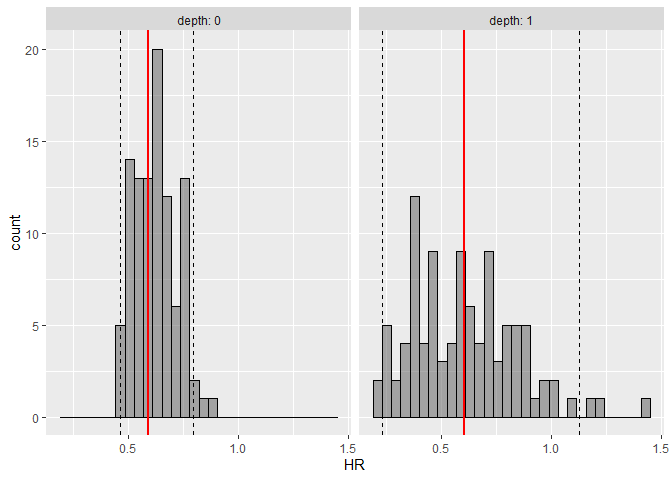Histogram of predicted hazard ratios, prediction intervals (dashed lines), and observed hazard ratios (red line), per depth groups

# rstanやrstanarm等をWindows上でソースからインストールする時の設定

ちなみに、コンピューターはWindows 7 or 10を使っているので、もしかしたらMac等では問題なく進むのかもしれません。

# RStanのWikiに書かれているステップを実行する

まずは下の2つのページに書かれているステップに従い、(1) C++ Toolchainのインストールと(2) Makevarsファイルの設定(2つ目のページの Configuration 項)を行います。

# g++のバージョンを確認する

R上で以下のコードを打ち、バージョンが4.9以降だったら問題ありません。

```system("g++ -v")
```

もしそうでない場合には、環境変数のPATHの頭に以下を追記します。

`C:\Rtools\bin;C:\Rtools\mingw_64\bin;C:\Rtools\mingw_32\bin;`

# Makevar.winと.Rprofileを編集する

## Makevars.win

Makevars.winの位置は`file.path(Sys.getenv("HOME"), ".R")`で、Windowsだと通常は `C:/Users/USERNAME/Documents/.R/`内にあります。 Makevars.win内の二行目のこれを

```CXX14 = g++ -m\$(WIN) -std=c++1y
```

```CXX14 = \$(BINPREF)g++ -m\$(WIN) -std=c++1y
```

## .Rprofile

.RprofileはSys.getenv("HOME")にあるはずですが、無ければ作成してください。上と同様に、Windowsだと通常は `C:/Users/USERNAME/Documents/`内に置かれます。 このファイル内に以下の行を追加してください。

```Sys.setenv(BINPREF = "C:/RTools/mingw_\$(WIN)/bin/")
```

*1:見たところ32ビット版のバイナリを作る時に64ビット版のg++を使ってしまっているようなので、PATH周りの問題かもしれません。

# Using purrr's map family functions in dplyr::mutate

`map` family functions of the `purrr` package are very useful for using non-vectorized functions in `dplyr::mutate` chain (see GitHub - jennybc/row-oriented-workflows: Row-oriented workflows in R with the tidyverse or https://www.jimhester.com/2018/04/12/vectorize/).
I encounter the needs for this especially when dealing with nested data frames.

One of the drawbacks is that name/input argument assignments become confusing when you want to use more than two columns of your data frames (and using `pmap` family) for the function of interest. This post first briefly review how `mutate` works in combination with `map` or `map2`, then provide two approaches to avoid confusions around name assignments when using `pmap`.

# How `mutate` works with vectorized functions

In most cases, the processes you want to do in `mutate` is vectorized and there is no need to use `map` family function. This works because the output from the function of interest (`c` in the example below) has the same length as the original data frame, and `mutate` only need to append one column to the data frame.

```library(tidyverse)

df0 <- tibble(a = 1:3, b = 4:6)

df0 %>% mutate(c = a + b)```

# Non-vectorized function with one or two input arguments (`map` or `map2`)

Imagine that we want to create a new column containing arithmetic progressions in each row [ref (in Japanese)]. Since `seq` function is not vectorized, we cannot directly use this in `mutate` chain.

```df1 <- tibble(a = c(1, 2), b = c(3, 6), c = c(8, 10))

df1 %>% mutate(d = seq(a, b))
# Error in mutate_impl(.data, dots) : Evaluation error: 'from' must be of length 1.```

Instead, we can use `map` family function here. `map` family function take list(s) as input arguments and apply the function of interest using each element of the given lists. Because each column of data frames in R is a list, `map` works very well in combination.

In this example, we want to provide two input arguments to the `seq` function, `from` and `to`. `map2` is the appropriate function for this.

```df2 <- df1 %>% mutate(d = map2(a, b, seq))

as.data.frame(df2)
#  a b  c             d
#1 1 3  8       1, 2, 3
#2 2 6 10 2, 3, 4, 5, 6```

The figure below shows how `map` function handles this process in `mutate` chain.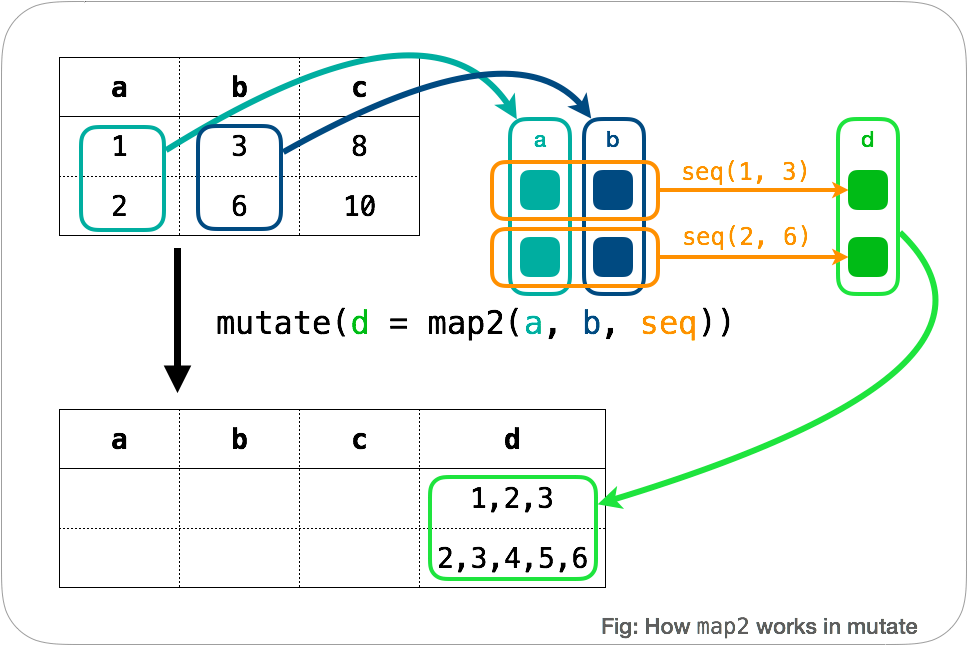Like you do with `map` function outside `mutate`, we can use `map_dbl` or `map_chr` to create columns with `double` or `character` types.

If we want to explicitly specify names of the argument, `.x` and `.y` can be used. See what happens with this:

```df2 <- mutate(df1, d = map2(a, b, ~seq(.y, .x)))

as.data.frame(df2)```

# Non-vectorized function with three or more input arguments (`pmap`)

Assignment of column names become confusing when using three or more columns, because we don't have shorthand like `.x` or `.y` any more. Let's take a look at the following example using `rnorm` function.

## Case example

Generate a list of random numbers for each row with `rnorm` function. Each row of the original data frame contain different value of `mean`, `sd`, `n`.

We will first prepare a data frame with columns corresponding to `mean`, `sd`, `n`, and apply `rnorm` function for each row using `pmap`. Each element of the new column `data` contains a vector of random samples *1. This type of structure is called as "nested data frames" and there are many resources on this, such as 25 Many models | R for Data Science.

## A simple case

If your data frame has the exact same names and numbers of columns to the input arguments of the function of interest, a simple syntax like the one below works *2.

```df4 <-
tribble(~mean, ~sd, ~n,
1,  0.03, 2,
10, 0.1,  4,
5,  0.1,  4)

df4.2 <-
df4 %>%
mutate(data = pmap(., rnorm))

as.data.frame(df4.2)```

One caution is that the syntax like the one below doesn't work. `pmap` thinks that you are calling `rnorm(df4\$n, df4\$mean, df4\$sd)` for each row, and each element of the new column contain three random samples from the same list of `mean` and `sd`. *3

`df4 %>% mutate(data = pmap(., ~rnorm(n, mean, sd))) %>% as.data.frame() # Wrong answer`

## Number of columns > Number of input arguments

In most cases, however, you will have more columns than the input arguments. `pmap` complains in this case, saying that you have unused argument.

```df5 <-
tribble(~mean, ~sd, ~dummy, ~n,
1,  0.03, "a", 2,
10, 0.1,  "b", 4,
5,  0.1,  "c", 4)

df5 %>% mutate(data = pmap(., rnorm))　 # Error```

There are two ways to avoid this error.

### Make a small list on the fly

The first method is to create a small list that only contains the necessary columns (Ref: Dplyr: Alternatives to rowwise - tidyverse - RStudio Community )

```df5.2 <-
df5 %>%
mutate(data = pmap(list(n=n, mean=mean, sd=sd), rnorm))

as.data.frame(df5.2)```

Here, `list(n=n, mean=mean, sd=sd)` create a new list with three vectors named `n`, `mean`, and `sd`, which serves the same purpose as the `df4` data frame in the above example.

Mind that if you don't give names to the elements of the new list, the order of the list items will be used to associate with input arguments of `rnorm`. My recommendation is to always assign names to the list elements.

```df5 %>% mutate(data = pmap(list(n, mean, sd), rnorm)) # Correct but not recommended
df5 %>% mutate(data = pmap(list(mean, sd, n), rnorm)) # Wrong answer```

### Use `...` to ignore unused columns

The second method is to absorb unused columns with `...` (Ref: Map over multiple inputs simultaneously. — map2 • purrr). A syntax like the one below works because `pmap` automatically associate names of the input list and names in `function()`. In other word, columns names of the data frame must match the variable names in the `function()`.

```df5.3 <-
df5 %>%
mutate(data = pmap(., function(n, mean, sd, ...) rnorm(n=n, mean=mean, sd=sd)))

as.data.frame(df5.3)```

Input arguments of `function()` and `rnorm()` are not automatically associated with names. It is recommended to explicitly associate input argument name for the function of interest (`rnorm` in this case).

```df5 %>% mutate(data = pmap(., function(n, mean, sd, ...) rnorm(n, mean, sd))) # Correct but not recommended
df5 %>% mutate(data = pmap(., function(n, mean, sd, ...) rnorm(mean, sd, n))) # Wrong answer
df5 %>% mutate(data = pmap(., function(mean, sd, n, ...) rnorm(mean, sd, n))) # Wrong answer```

A syntax like the one below gives unexpected outputs, as you saw in the `df4` example.

`df5 %>% mutate(data = pmap(., function(...) rnorm(n=n, mean=mean, sd=sd))) # Wrong answer`

## Column names are different from the input argument names

You can use either of the two approaches above.

```df6 <-
tribble(~mean1, ~sd1, ~dummy, ~n1,
1,  0.03, "a", 2,
10, 0.1,  "b", 4,
5,  0.1,  "c", 4)

df6.2 <-
df6 %>% mutate(data = pmap(list(mean=mean1, sd=sd1, n=n1), rnorm))
as.data.frame(df6.2)

df6.3 <-
df6 %>% mutate(data = pmap(., function(n1, mean1, sd1, ...) rnorm(n=n1, mean=mean1, sd=sd1)))
as.data.frame(df6.3)
```

*1:In the examples below (and above), we further use as.data.frame function to exposure actual numbers of vectors

*2:This works even if the order of the columns is different from the order of input arguments

*3:This happens because rnorm is actually vectorized. See ?rnorm: If length(n) > 1, the length is taken to be the number required.

# dplyr::mutate内でベクトル化されていない関数を使う - purrr::pmapでのカラム名の対応付け

Rのデータフレームの各行に対して処理を行いたいが、関数をベクトル化することが難しい場合*1には、下で簡単に書くように`mutate``map`を組み合わせると非常に便利です。しかし、`map`内で定義する関数の入力値が3つ以上になると話が少しややこしくなります。納得するまで結構混乱したので、自分の備忘録も兼ねて記事にしておくことにしました。

# 通常の`mutate`を使った処理

```library(tidyverse)

df0 <- tibble(a = 1:3, b = 4:6)

df0 %>% mutate(c = a + b)```

# 各行について別々に処理、入力値が2つまでの場合

こちらにあるように、データフレームの各行に対して等差数列を作成する例を考えてみます。 このとき、`seq`関数はベクトル化されていないため、以下のように書くとエラーになります。

```df1 <- tibble(a = c(1, 2), b = c(3, 6), c = c(8, 10))

df %>% mutate(d = seq(x, y))
# Error in mutate_impl(.data, dots) : Evaluation error: 'from' must be of length 1.```

ここで`map`系関数の出番です。
`map`系関数は、リストを引数とし、リスト内の各要素に対して与えられた関数を適用した結果を返すという働きを持っています。 Rのデータフレームは各列がそれぞれリストとなっているので、`map`系巻数と相性が良くなっています。

```df2 <- df1 %>% mutate(d = map2(a, b, seq))

print(df2\$d)```もちろん、使用したい処理が一つの引数のみを取る場合は`map`を使えばいいですし、作成する列を`double``character`型にしたければ`map_dbl``map_chr`などを使います。 また、`map``map2`では、関数内で明示的に引数を指定したい場合、`.x``.y`を使います。例えば、上の例で`df2 <- mutate(df1, d = map2(a, b, ~seq(.y, .x)))`を実行してみてください。

# 各行について別々に処理、入力値が3つ以上の場合

この様に`map`系関数を使うと、ベクトル化されていない処理を`mutate`内で簡潔に書くことが出来ます。 3つ以上のカラムを使用したい場合、同様に`pmap`を使うことになるのですが、カラム名と関数の引数名の対応付けが少し複雑になります。

## 使用する例

ここではまず、`mean``sd``n`に対応する3つのカラムを持ったデータフレームを作成し、各行ごとに`rnorm`関数を適用して乱数を発生させています。新しく生成されたカラム`data`には、各行ごとに乱数値を保持したベクトルが格納されます。

ネスト化周りに関してはR4DSなど色々と解説があると思うので、馴染みのない方はそちらも参考にしてみてください。

## 単純な例

データフレーム内のカラムが、pmap内で使用する関数の引数と個数・名前共に一致する場合にのみ、以下のような簡潔な文法が通じます。

```df4 <-
tribble(~mean, ~sd, ~n,
1,  0.03, 2,
10, 0.1,  4,
5,  0.1,  4)

df4 %>% mutate(data = pmap(., rnorm)) %>% unnest()```

ちなみに、下のような書き方だと、rnorm内の入力は`df4\$n` `df4\$mean` `df4\$sd`であると見做されてしまい、想定していない結果となってしまいます。

`df4 %>% mutate(data = pmap(., ~rnorm(n, mean, sd))) %>% unnest() # Wrong answer`

## 余分なカラムが含まれている場合

```df5 <-
tribble(~mean, ~sd, ~dummy, ~n,
1,  0.03, "a", 2,
10, 0.1,  "b", 4,
5,  0.1,  "c", 4)

df5 %>% mutate(data = pmap(., rnorm))　 # Error```

これを回避するには、2つの方法があります。

### 小さいリストを作ってしまう

1つ目は、pmapの第一引数を`df4`のようなリストにその場で変えてしまう方法です。(参照: Dplyr: Alternatives to rowwise - tidyverse - RStudio Community )

`df5 %>% mutate(data = pmap(list(n=n, mean=mean, sd=sd), rnorm)) %>% unnest()`

```df5 %>% mutate(data = pmap(list(n, mean, sd), rnorm)) %>% unnest() # Correct but not recommended
df5 %>% mutate(data = pmap(list(mean, sd, n), rnorm)) %>% unnest() # Wrong answer```

### `...` で未使用変数を受け流す

2つ目は、`pmap`への入力ではデータフレームをそのまま渡す一方で、関数宣言の所で未使用の変数を受け流す方法です(参照: Map over multiple inputs simultaneously. — map2 • purrr, "Use `...` to absorb unused components of input list .l")。
`pmap`が、入力したリスト名と関数の引数名を自動で照合してくれる性質を利用しているので、`function()`内の変数名とデータフレームのカラム名が一致する必要があります。

`df5 %>% mutate(data = pmap(., function(n, mean, sd, ...) rnorm(n=n, mean=mean, sd=sd))) %>% unnest()`

ただしもちろん、普段関数を使う際と同様に、引数を名前で紐付けないと誤った結果となります。

```df5 %>% mutate(data = pmap(., function(n, mean, sd, ...) rnorm(n, mean, sd))) %>% unnest() # Correct but not recommended
df5 %>% mutate(data = pmap(., function(n, mean, sd, ...) rnorm(mean, sd, n))) %>% unnest() # Wrong answer
df5 %>% mutate(data = pmap(., function(mean, sd, n, ...) rnorm(mean, sd, n))) %>% unnest() # Wrong answer```

`df5 %>% mutate(data = pmap(., function(...) rnorm(n=n, mean=mean, sd=sd))) %>% unnest() # Wrong answer`

## カラム名がpmap内で使用する関数の引数名と一致しない場合

```df6 <-
tribble(~mean1, ~sd1, ~dummy, ~n1,
1,  0.03, "a", 2,
10, 0.1,  "b", 4,
5,  0.1,  "c", 4)

df6 %>% mutate(data = pmap(list(mean=mean1, sd=sd1, n=n1), rnorm)) %>% unnest()
df6 %>% mutate(data = pmap(., function(n1, mean1, sd1, ...) rnorm(n=n1, mean=mean1, sd=sd1))) %>% unnest()
```

# map系関数の使い方について参考になるサイト

*1:特にnested dafa frameを扱っているときに頻発します

# Pharmacometrics解析のためのRStanへのTorstenライブラリの導入

Metrum Research Groupが開発しているTorstenライブラリは、Stan上でPharmacometrics解析を行うための拡張ライブラリです。 BaseのStanパッケージに比べ、以下の拡張がなされています。

• NONMEMフォーマットのデータセットの読み込みとイベントスケジュール(薬剤投与、測定)の処理
• 標準薬物動態ライブラリの搭載 (NONMEMのPREDPPのようなもの)
• 線形1-/2-コンパートメントモデル+一次吸収の解析解
• 汎用ODEモデル (Runge KuttaもしくはBDF)

この記事では、TorstenライブラリをRStanに導入する方法を解説し、一例として2-コンパートメントモデルを使ってみます。

### インストール

#### 手順

R上で以下のコマンドを入力するとインストールが行われます。RStanライブラリのヘッダーを置き換えてコンパイルをし直すようなので、インストールに比較的時間がかかります。

```devtools::install_github('metrumresearchgroup/TorstenHeaders')
```

### 使用例

こちらもMetrumによるGitHubリポジトリに、Torstenを使用した幾つかのモデルの例が載っています。今回はこのうちの2-コンパートメントモデルの例を使用してみます。
https://github.com/metrumresearchgroup/example-models
Torstenのマニュアルもこのリポジトリに含まれています(PDFへのリンク)。

はじめに、今回使用するライブラリを読み込んでおき、RStanの設定もしてしまいます。

```library(tidyverse)
library(ggmcmc)
library(rstan)

rstan_options(auto_write=T)
options(mc.cores=parallel::detectCores())
```

#### データセット

```data <- rstan::read_rdump("TwoCptModel.data.R")
```

• amt: 薬物の投与量
• ii: 投与間隔
• cmt: イベントが発生するコンパートメントのインデックス (1は吸収コンパートメント、2は中心コンパートメント)
• cObs: 測定値
• evid: 測定の場合は0、投与の場合は1に対応するインデックス
• iObs: 全イベントのうち測定に対応する行のインデックス
• nObs: 測定データの個数
• nt: 全イベントの個数
• rate: 定速静注の速度(今回は使用なし)
• ss: 定常状態から計算の開始(今回は使用なし)
• time: 各イベントが発生した時間

となっています。

https://github.com/metrumresearchgroup/example-models/blob/torsten-0.83/TwoCptModel/TwoCptModelSimulation.R

#### Stanモデル

https://github.com/metrumresearchgroup/example-models/blob/torsten-0.83/TwoCptModel/TwoCptModel.stan

```  // PKModelTwoCpt takes in the NONMEM data, followed by the parameter
// arrays and returns a matrix with the predicted amount in each
// compartment at each event.
x = PKModelTwoCpt(time, amt, rate, ii, evid, cmt, addl, ss,
theta, biovar, tlag);```

timeからssまではデータセットの中身をそのまま渡しています。 thetaがパラメータ(CL, Q, V1, V2, ka)を含む配列、biovarとtlagは追加のパラメータです。それぞれ、

• CL: 中心コンパートメントからの消失クリアランス
• Q: 中心コンパートメントと末梢コンパートメント間のクリアランス
• V1: 中心コンパートメントの分布容積
• V2: 末梢コンパートメントの分布容積
• ka: 吸収速度定数
• biovar: 各コンパートメントへのバイオアベイラビリティ
• tlag: 各コンパートメントへの投与ラグタイム

を表します。

この関数を走らせると、渡したtimeにおける全コンパートメントの薬物量がmatrixとして返されます。

#### Rスクリプト

ただ、色々と不可欠で無いものが入っているので、最小限の要素のみ残したものを以下に記載します。 ここでは、Stanモデルファイルがworking directlyに保存されていると仮定しています。また、データファイルは上記のコードを使って読み込み済みと仮定しています。

```## create initial estimates
init <- function() {
list(CL = exp(rnorm(1, log(10), 0.2)),
Q = exp(rnorm(1, log(15), 0.2)),
V1 = exp(rnorm(1, log(35), 0.2)),
V2 =  exp(rnorm(1, log(105), 0.2)),
ka = exp(rnorm(1, log(2), 0.2)),
sigma = runif(1, 0.5, 2))
}

## run Stan
fit <- stan(file = "TwoCptModel.stan",
data = data,
init = init)
```

```fit.param <- ggs(fit)
list.param <- c("CL", "Q", "V1", "V2", "ka")

fit.param.plot <-
fit.param %>% filter(Parameter %in% list.param)

ggs_density(fit.param.plot)
ggs_traceplot(fit.param.plot)
ggs_autocorrelation(fit.param.plot)
ggs_Rhat(fit.param.plot)
```

```mcmc.sample <- rstan::extract(fit)

obs.pred.interval <-
mcmc.sample\$cObsPred %>%
apply(MARGIN=2,quantile, prob=c(0.05, 0.5, 0.95)) %>%
t() %>%
tbl_df()

obs.pred.interval %>%
mutate(Time = data\$time[data\$iObs],
cObs = data\$cObs) %>%
ggplot(aes(Time, `50%`)) +
geom_line() +
geom_ribbon(aes(ymin=`5%`, ymax=`95%`),alpha=0.1) +
geom_point(aes(y=cObs)) +
scale_x_continuous(breaks=seq(0, 200, by=24)) +
xlab("Time (hour)") +
ylab("Concentration")
```

### 参照したリンク先のまとめ

*1:省略できるよう改良を計画しているようです。

*2:RとStanコードを改良することで、測定点以外の時間についてもシミュレーション結果を表示することが可能です。そのうちそれについて記事を書くかもしれません。

# Stan & POPPK (4): Covariateの影響を考慮した解析

Stan & POPPK (3): 多変量正規分布を用いたパラメータ間の相関まで含めた解析 - yoshidk6’s blog の続きです。パラメータの個人間変動を生む要因を分析してモデルに組み込みます。

### パラメータの個人間変動を生じる因子の探索

```# 推定パラメータ値の抽出
fit.CLi <-
summary(fit.stan, pars = c("CLi"))\$summary %>%
tbl_df() %>%
select(mean)
fit.VDi <-
summary(fit.stan, pars = c("VDi"))\$summary %>%
tbl_df() %>%
select(mean)

fit.indiv.params <-
bind_cols(tibble(ID=1:nrow(fit.CLi)),
fit.CLi %>% rename(CL=mean),
fit.VDi %>% rename(VD=mean))

fit.indiv.params %>%
ggplot(aes(CL,VD)) +
geom_point() +
geom_smooth(method="lm")

# 体重・性別データと結合し、パラメータ値との相関をプロット

fit.indiv.params.cov <-
full_join(fit.indiv.params, data.cov)

fit.indiv.params.cov %>%
gather(Parameter, Value, CL, VD) %>%
ggplot(aes(WT,Value)) +
geom_point() +
facet_wrap(~Parameter, scales="free") +
geom_smooth(method="lm")

fit.indiv.params.cov %>%
gather(Parameter, Value, CL, VD) %>%
ggplot(aes(factor(SEX),Value)) +
geom_boxplot() +
facet_wrap(~Parameter, scales="free")
```

### 解析

#### Rコード

```library(tidyverse)
library(rstan)
library(ggmcmc)

rstan_options(auto_write=T)
options(mc.cores=parallel::detectCores())

init <- function(){
list(KA = exp(rnorm(1, log(0.5), 0.2)),
CL = exp(rnorm(1, log(0.5), 0.2)),
VD = exp(rnorm(1, log(5), 0.2)),
sigma = exp(rnorm(2, log(0.2), 0.5)),
rho = diag(2),
s_Y = runif(1, 0.5, 2),
WTCL= rnorm(1, 0.5, 1),
WTVD= rnorm(1, 0.5, 1),
CLVDiLog=matrix(rep(log(c(0.5,5)), ea = nrow(data.subj)),
nrow = nrow(data.subj)))
}

data <-
list(N    = nrow(data.pk),
N_ID = nrow(data.subj),
ID   = data.pk\$ID,
TIME = data.pk\$TIME,
DOSE = data.subj\$DOSE,
WT   = data.cov\$WT,
WTMED= median(data.cov\$WT),
Y    = data.pk\$CONC)

nChains <- 4
nPost <- 1000 ## Number of post-burn-in samples per chain after thinning
nBurn <- 1000 ## Number of burn-in samples per chain after thinning
nThin <- 10

nIter <- (nPost + nBurn) * nThin
nBurnin <- nBurn * nThin

fit.stan <-
stan(file = "mod_04_cov.stan",
data = data, init=init,
iter = nIter,
warmup = nBurnin,
thin = nThin,
chains = nChains,
```

#### 結果の評価

WTCLはおおよそ0.75、WTVDはおおよそ1.07と推定されました。体重が1.5倍になるとCLとVDはそれぞれ1.35倍、1.5倍になるということになり、比較的大きな影響を持っていると言えます。

# Stan & POPPK (3): 多変量正規分布を用いたパラメータ間の相関まで含めた解析

Stan & POPPK (2): 階層モデルを用いた複数被験者の薬物動態解析 - yoshidk6’s blog の続きです。前回使用したモデルを拡張して、パラメータ間の相関も含めた解析を行います。

### 解析

#### Stanモデル

モデル構造は前回とほぼ同じですが、多変量正規分布を扱うための変更を行っています。
Stan超初心者入門 の114ページ目から詳しい説明が載っています。

#### Rコード

```library(tidyverse)
library(rstan)
library(ggmcmc)

rstan_options(auto_write=T)
options(mc.cores=parallel::detectCores())

init <- function(){
list(KA = exp(rnorm(1, log(0.5), 0.2)),
CL = exp(rnorm(1, log(0.5), 0.2)),
VD = exp(rnorm(1, log(5), 0.2)),
sigma = exp(rnorm(2, log(0.2), 0.5)),
rho = diag(2),
s_Y = runif(1, 0.5, 2),
CLVDiLog=matrix(rep(log(c(0.5,5)), ea = nrow(data.subj)),
nrow = nrow(data.subj)))
}

data <-
list(N    = nrow(data.pk),
N_ID = nrow(data.subj),
ID   = data.pk\$ID,
TIME = data.pk\$TIME,
DOSE = data.subj\$DOSE,
Y    = data.pk\$CONC)

fit.stan <-
stan(file = "mod_03_multinorm.stan",
data = data, init=init)
```

#### 結果の評価